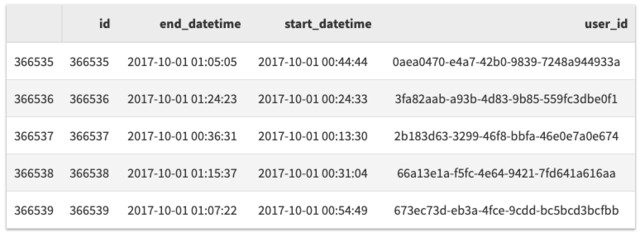【翻译】适用于产品经理的 Python 课程（二）

Posted by zhang on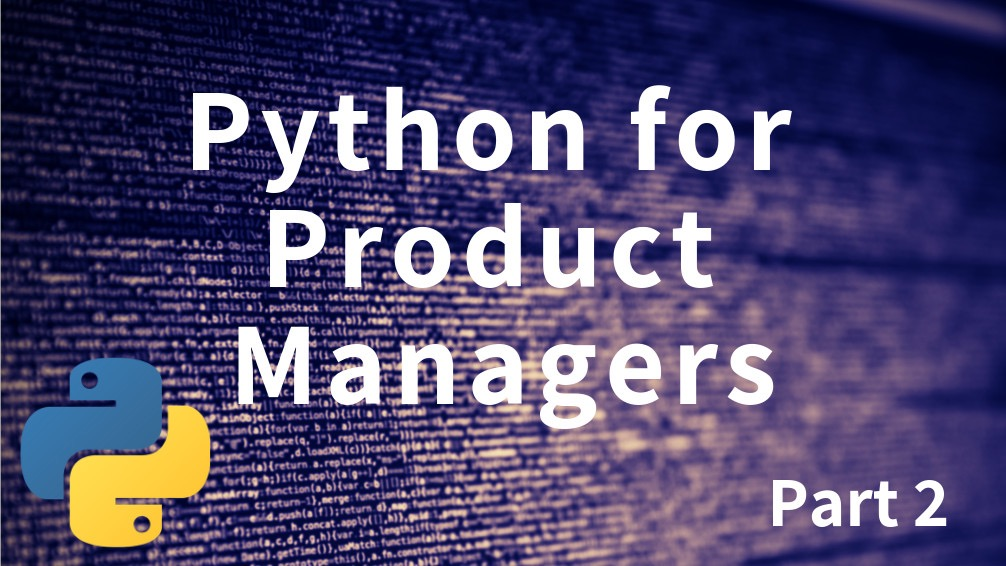## 导入 Pandas

``````import pandas as pd
``````

``````# 在 Jupyter Notebooks 中内嵌显示图形和图标
%matplotlib inline

# 导入一些库
import pandas as pd
import matplotlib
import matplotlib.pyplot as plt

# 设置一些默认的绘图样式使图标看起来更 nice
matplotlib.rcParams['figure.figsize'] = (20.0, 10.0)
plt.style.use('bmh')
``````

## 了解 Pandas DataFrame

Pandas 中的主要数据结构是 DataFrame，你可以把它视作 Excel 表格或者数据库表。他是一个包含列和行的数据集合。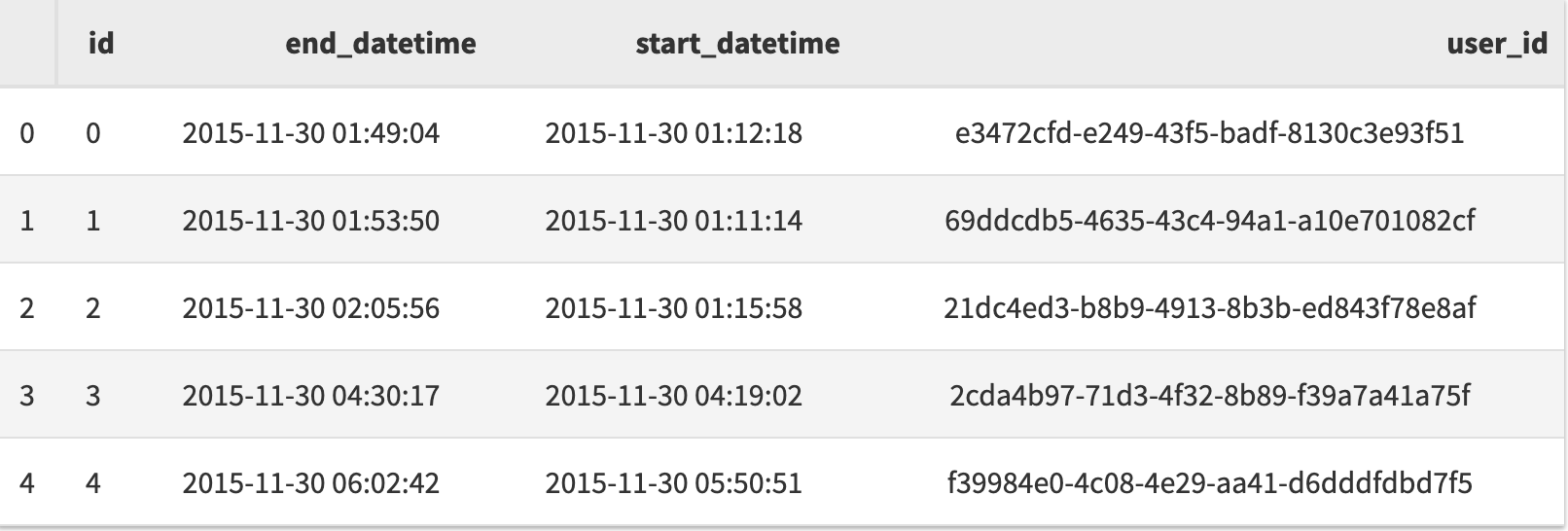## 加载数据

### 通过文本文件

``````##从 0.21.0开始不推荐使用 from_csv，开始使用 read_csv，下边都是以 read_csv 作为例子
df = pd.read_csv('my_csv_file.csv')
``````

sep-定义文件中使用的列分隔符（例如：\t 代表 tab,|），默认值为逗号（,）;
parse_dates-指定包含日期的列或者列数组 ，pandas 将尝试转换列中数据的数据类型为 date time – 这将更好轻松地进行基于日期的分组（例如按年份或月份分组）；
header=None- 如果数据文件没有表头，请使用此选项。name 参数用于设置每列的名称，例如`names=["col1", "col2", "col3"]`

``````df = pd.read_csv('my_csv_file.txt', sep='\t' header=None, names=['id', 'end_datetime', 'start_datetime', 'user_id'], parse_dates=['end_datetime','start_datetime'])
``````

### 来自数据库

``````import mysql.connector

# 创建一个数据库连接
conn = mysql.connector.connect(host='hostname',database='db_name',user='user_name',password='xxxxxx')

# 定义将提取数据的 SQL 查询语句
sql = "SELECT * FROM sessions"

# 创建包含查询结果的 DataFrame
df = pd.read_sql(sql, conn, parse_dates=['start_datetime', 'end_datetime'])
df.head()
``````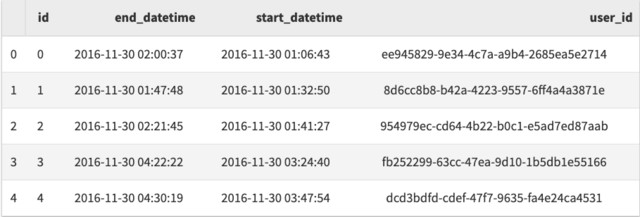1. 如果你了解 SQL 语句，则可以在数据加载到 Python 之前进行一些过滤和数据操作。
2. 假如你的数据库经常更新，它很容易刷新并重复你的分析，以获取关于你的数据的最新分析结果。

### 带参数查询

``````
# 使用参数占位符定义 SQL
sql = "SELECT * FROM sessions WHERE YEAR(start_datetime) = %s"

# 使用提供的参数提取数据
df = pd.read_sql(sql, conn, parse_dates=['start_datetime', 'end_datetime'], params=)
df.head()
``````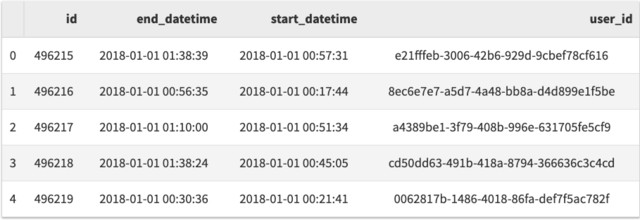### 来自现有的 Python 变量

``````my_dict = [
{'id': 0, 'name':'Bob', 'age':64},
{'id': 1, 'name':'Sally', 'age':61},
{'id': 2, 'name':'June', 'age':32},
{'id': 3, 'name':'Nish', 'age':41},
{'id': 4, 'name':'Lexi', 'age':25}
]

df = pd.DataFrame.from_dict(my_dict)
df.head()
``````## DataFrame 分类

``````df.sort_values('start_datetime').head(n=3)
````````````df.sort_values('start_datetime', ascending=False).head(n=3)
``````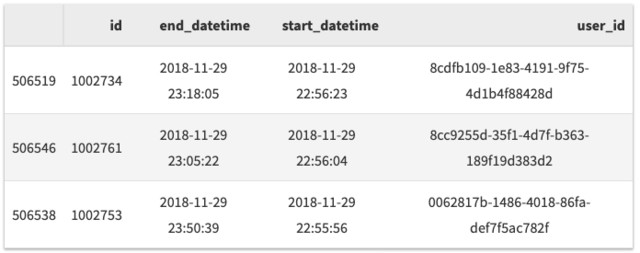``````df.sort_values(['start_datetime', 'user_id']).head(n=3)
``````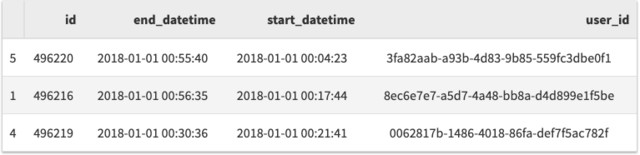## 过滤数据

``````df[df['user_id']=='8ec6e7e7-a5d7-4a48-bb8a-d4d899e1f5be'].head()
``````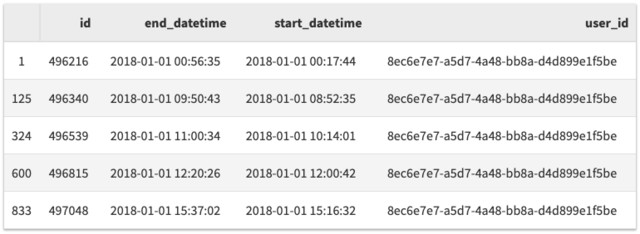``````df[(df['start_datetime'].dt.month==10) & (df['start_datetime'].dt.year >= 2017)].head()
``````